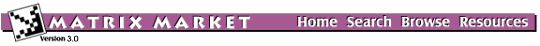## KAHAN: Upper trapezoidal matrix for testing condition and rank estimation

### from the MMDELI

Matrix Generator KAHAN
Source: W. Kahan
Discipline: numerical linear algebra
Language: Java applet
Output format: Matrix Market format

This is a Java applet which generates the Kahan matrix, an upper trapezoidal matrix that has some interesting properties regarding estimation of condition and rank. It is defined as S*C + P, where P = PERTURB*EPS*diag( N, N-1, ..., 1), S = diag( 1, s, s2, ..., sN-1), EPS is the machine epsilon, s = sin(THETA), c = cos(THETA), and C is the upper triangular matrix with 1s on the diagonal and -c on the off-diagonals. If N > M zero rows are appended. If N < M the last column is replicated.

The diagonal is perturbed to ensure that the QR factorization with column pivoting does not interchange columns in the presence of rounding errors. For example, PERT = 25 and THETA = 1.2, ensures that no interchanges are necessary up to at least N = 90 in IEEE arithmetic.

The inverse of this matrix is known explicitly and can be computed here (see Higham, SIAM Review, 1987, p. 588 for details). The diagonal perturbation was suggested by Christian Bischof.

This generator was developed by Bruce Miller of NIST based on Nick Higham's Test Matrix Toolbox for MATLAB.

Parameters:

 N number of rows M number of columns THETA angle (0 < THETA < PI) PERTURB diagonal perturbation parameter INVERSE switch to compute inverse matrix (if it exists)

## Links:

The Matrix Market is a service of the Mathematical and Computational Sciences Division / Information Technology Laboratory / National Institute of Standards and Technology.

[ Home ] [ Search ] [ Browse ] [ Resources ]

Last change in this page: Wed Sep 22 13:34:22 US/Eastern 2004 [Comments: ]